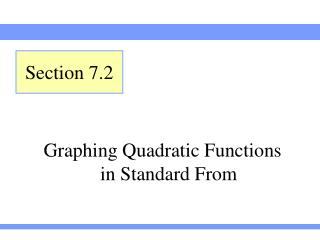DownloadDownload PresentationGraphing Quadratic Functions in Standard From

# Graphing Quadratic Functions in Standard From

Télécharger la présentation## Graphing Quadratic Functions in Standard From

- - - - - - - - - - - - - - - - - - - - - - - - - - - E N D - - - - - - - - - - - - - - - - - - - - - - - - - - -
##### Presentation Transcript

1. Section 7.2 Graphing Quadratic Functions in Standard From

2. Example A fireworks shell is launched into the air. The shell’s height (in feet) h = f (t) at time t seconds is modeled well by the function When should the shell explode so that it goes off at the maximum height? What is that height? Section 7.2 Slide 2 Minimum of Maximum Value Finding a Maximum Value of a Quantity

3. Solution a = –16, which is negative Parabola opens downwards So, there is a maximum t-coordinate is: h-coordinate is: So, the vertex is (6.25, 626) Thus, maximum height is 626 feet at 6.25 seconds and should explode then Section 7.2 Slide 3 Minimum of Maximum Value Finding a Maximum Value of a Quantity